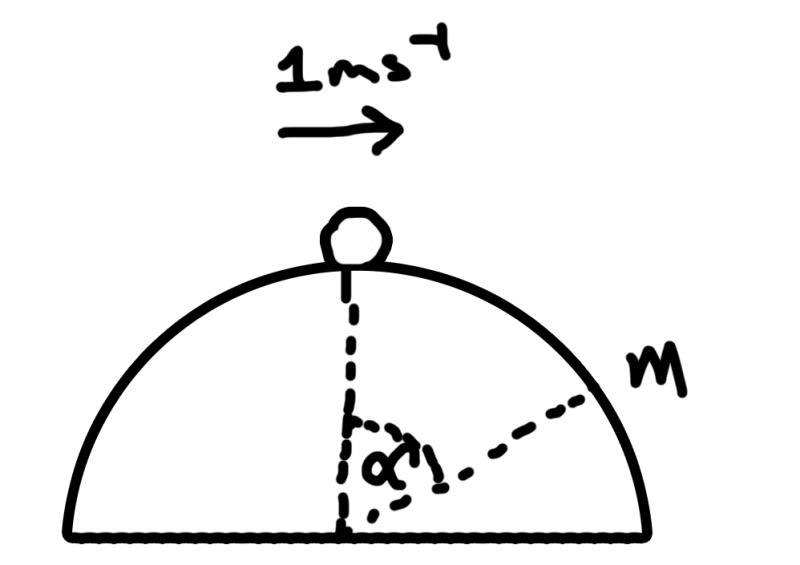# Vertical circles

RJWills

## Homework Statement

F=Force, v=Velocity, a=acceleration m=mass (0.01kg) R=Normal Reaction to surface

Hi, can anyone help, I keep getting the wrong answer on this question and it is really annoying me. Here is a picture of the diagram:"A Marble of mass 0.01kg is on top of a smooth hemispherical bowl and given an initial speed of 1 m/s. The bowl has a radius of 0.5m and centre o.
a) The marble descends 0.1m, find the speed. got this part correct and figured out that v^2=1+0.2g

b) "Find the normal reaction of the bowl at this point"

## Homework Equations

I have a solution for Velocity^2=1+0.2g

## The Attempt at a Solution

So I have v^2=1+0.2g, now trying to resolve in the direction of R using F=ma I got:
R-0.01gcos(alpha)=m x (v^2/r)
=>R=0.01x(1+0.2g/0.5)
Now, trying to find alpha I thought of the height raise as 0.4m and the radius 0.5 to create a right angled triangle, getting cos(alpha)=0.4/0.5, and subsequently alpha to equal 36.86... deg.

When I sub this is my value that I get for R=0.1376, however in the book it says it should = 0.0192.

I have tried re doing it from scratch twice, faffing about with different bits and I just cannot get it right :/

Mentor
So I have v^2=1+0.2g, now trying to resolve in the direction of R using F=ma I got:
R-0.01gcos(alpha)=m x (v^2/r)
This looks OK.
=>R=0.01x(1+0.2g/0.5)
What happened to the 0.01gcos(alpha) term? (But it looks like you include that term in your calculation.)
Now, trying to find alpha I thought of the height raise as 0.4m and the radius 0.5 to create a right angled triangle, getting cos(alpha)=0.4/0.5, and subsequently alpha to equal 36.86... deg.
OK.

When I sub this is my value that I get for R=0.1376, however in the book it says it should = 0.0192.
I get the same answer that you do.

Ah... Here's the problem: The marble's rolling down the inside of a concave bowl. Your diagram is not correct.

RJWills
This looks OK.

What happened to the 0.01gcos(alpha) term? (But it looks like you include that term in your calculation.)

I get the same answer that you do.

Ah... Here's the problem: The marble's rolling down the inside of a concave bowl. Your diagram is not correct.

As you say, I have included it in my working, i just miss typed what I have written on paper.
The diagram is included in the textbook question. Still really confused and I cannot see how to solve this.

Edit: HURRAY SOLVED IT! I was thinking of it as R-0.01g= xyz where as it should be 0.01g-R!

Thanks :)

Mentor
As you say, I have included it in my working, i just miss typed what I have written on paper.
Good.
The diagram is included in the textbook question. Still really confused and I cannot see how to solve this.
Yes, my bad. I was making the same mistake as you!You have the direction of the acceleration and the reaction force wrong.

Mentor
Edit: HURRAY SOLVED IT! I was thinking of it as R-0.01g= xyz where as it should be 0.01g-R!
Yay! Sorry for not spotting that earlier. :uhh: